## on output format from solve with exponential...

Why Maple likes to extract exp() outside the sqrt when its argument has minus sign vs. not?  Compare the following

restart;
eq2 := ln(2*u^2 + u - 1) = -c - 2*ln(x);
sol:=[solve(eq2,u)]:
simplify(sol)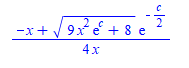and

eq2 := ln(2*u^2 + u - 1) = c - 2*ln(x);
sol:=[solve(eq2,u)]:
simplify(sol)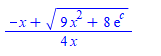I like the above much better than the first one. Mathematica keeps both same form (i.e. keeps the exp() inside):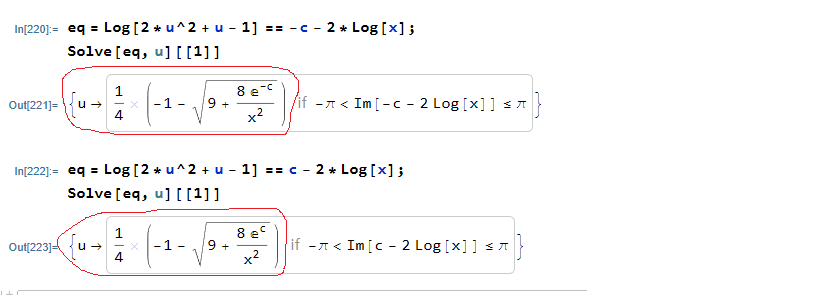Maple's answers are correct ofcourse, I just do not understand the logic why when there is a minus sign on it likes to format it differently as shown.

Is there a way to make not do that?

## Exporting 3D Plot in Vector Graphics Format...

I am trying to export a 3D plot from Maple (2021) in a vector format that can be imported into Adobe Illustrator without too much trouble. The graphic artist says the PDF files are not useful (“at the base of every PDF is an image file, inside layers and layers of clipping paths; not helpful”). “SVG does not appear to produce usable vectors – well, maybe not, but the one you sent me was super difficult to wrangle”. She had hoped that EPS would be useful, but she was unable to open the EPS file I created (not enough memory).

Here is the example that I have been using as I've asked others for help with this question.

restart;
with(plots);
with(plottools);

T := torus([0, 0, 0], 1/2, 1/2);
C0 := spacecurve([cos(t), sin(t), 0], t = 0 .. 2*Pi, color = red, thickness = 9);
C1 := spacecurve([0.5*cos(t), 0.5*sin(t), 0.5], t = 0 .. 2*Pi, color = red, thickness = 9);
C2 := spacecurve([0.5*(1 + cos(t)), 0, 0.5*sin(t)], t = 0 .. Pi, color = blue, thickness = 9);
C3 := spacecurve([0.5*(-1 + cos(t)), 0, 0.5*sin(t)], t = 0 .. Pi, color = blue, thickness = 9);
Fig9510 := display([T, C0, C1, C2], view = [DEFAULT, DEFAULT, 0 .. 1/2], scaling = constrained, transparency = 0.75);

Direct links to the worksheet and the PDF, EPS, and SVG files on DropBox are provide below in the hope that something will be useful to somebody. (MaplePrimes links are provided for the MW and PDF files are also provided - but EPS and SVG files are not permitted.)

1. 3DPlotExample.mw: https://www.dropbox.com/s/mx1hg1ntuqihnu3/3DPlotExample.mw?dl=0
2. Fig9510.pdf: https://www.dropbox.com/s/bnzfqrh060gw84g/Fig9510.pdf?dl=0
3. Fig9510.eps: https://www.dropbox.com/s/h9bjyn0uwm8w5ct/Fig9510.eps?dl=0
4. Fig9510.svg: https://www.dropbox.com/s/u2xfntnqvbhu810/Fig9510.svg?dl=0

This question has been asked for more than ten years. The best solution to has been to use the command-line-interface version of Maple.

1. Is the command-line interface still the best/only option for vector-based graphics output?
2. If so, how do i access the command-line version of Maple 2021 on MacOS?

## How to Use Nonexecutable math in documents.?...

The attached worksheet is a stripped down version of some notes I am creating concerning the solution of vector equations in geometric algebra.  For the purposes of explanation it is best to show the math in its conventional typeset form before showing how to solve the equations in maple. The non-excutable math is in the text which is contained in a paragraph inserted before the execution group. In the first instance, the typesetting engine seems content to leave the text alone, but in the second case (which I created in the same way) it insists on parsing the equations and generating errors. I have tried repeatedly to make the second set of equations non-executable (since in maple terms they are nonsense) to no avail.
Can anyone suggest how I can use maple's text features to make the explanatory text inert.

Note that I have included the calls to my geometric algebra module in case they have some relevance to the problem, but they will not execute without the module.

Sample worksheet:  nonexecmatherror.mw

## Error attempting to install Physics Package Update...

When I click on the "install" option for the update to the Physics Package, get the following error message:

Fetching package "Physics Updates" from MapleCloud...
ID: 5137472255164416
Version: 988
URL: https://maple.cloud

An error occurred, the package was not installed:

## Error, (in SumTools:-DefiniteSum:-ClosedForm) nume...

In Maple 2021.1

restart;
int(cos(3*x)/(-(-1+8*cos(x)^2)^(1/2)+(3*cos(x)^2-sin(x)^2)^(1/2)),x)


gives

Error, (in SumTools:-DefiniteSum:-ClosedForm) numeric exception: division by zero

But in Maple 2020.2 it works OK giving an answer. (A very long one)

3/4*arcsin(2/3*sin(x)*3^(1/2))-3/4*arctan(sin(x)/(-1+4*cos(x)^2)^(1/2))-3/4*arctan(sin(x)/(-1+8*cos(x)^2)^(1/2
))+5/8*arcsin(2/7*sin(x)*14^(1/2))*2^(1/2)-1/2*sin(x)*(-1+4*cos(x)^2)^(1/2)-1/2*sin(x)*(-1+8*cos(x)^2)^(1/2)

Is this a known issue? I know Maple int has went some changes and improvements in Maple 2021 from the release notes. May be this was caused by some of these changes?

https://www.maplesoft.com/products/maple/new_features/

• Integration has been enhanced with improved algorithms for indefinite integration, and the ability to easily specify which integration method should be used and to compare the results from different methods."

Maple 2021.1 on windows 10

update July 2, 2021

These are additional failed integration in 2021.1 that throw exceptions now. no errors in 2020.2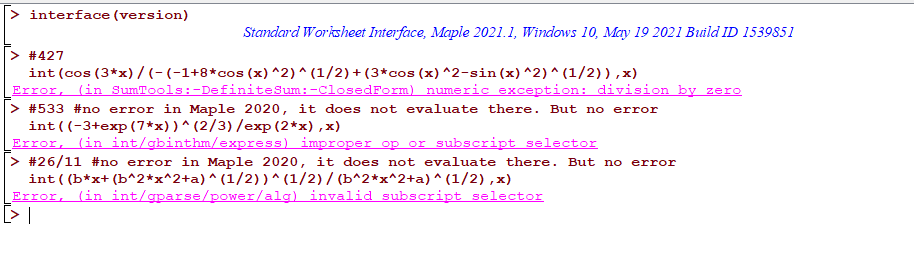#427
int(cos(3*x)/(-(-1+8*cos(x)^2)^(1/2)+(3*cos(x)^2-sin(x)^2)^(1/2)),x)

#533 #no error in Maple 2020, it does not evaluate there. But no error
int((-3+exp(7*x))^(2/3)/exp(2*x),x)

#26/11 #no error in Maple 2020, it does not evaluate there. But no error
int((b*x+(b^2*x^2+a)^(1/2))^(1/2)/(b^2*x^2+a)^(1/2),x)


## why dfieldplot and phaseportrait and DEplot not ac...

dsolve accept system of first order ode's in the form x'=A x, where x' is vector, A is matrix of coefficients and x are the dependent variables. This is convenient since one does not have to convert things to a list.

But  dfieldplot and phaseportrait and DEplot do not accept this form. One must convert things to list first.

Here is an example

restart;
sys:=Vector([diff(x(t),t),diff(y(t),t)]) = Matrix([[1,2],[0,3]]).Vector([x(t),y(t)]);
dsolve(sys)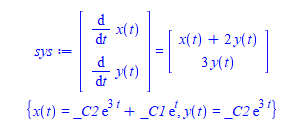But

DEtools:-dfieldplot(sys,[x(t),y(t)],t=0..4,x=-4..4, y=-4..4);


Error, (in DEtools/dfieldplot) system must have same number of dependent variables as DE's.

workaround is to write the system as list

new_sys:=[diff(x(t), t)=x(t) + 2*y(t),diff(y(t), t)=3*y(t)];
DEtools:-dfieldplot(new_sys,[x(t),y(t)],t=0..4,x=-4..4, y=-4..4);


I know one can automate the conversion. But still, it would be better if dfieldplot would accept sys as dsolve did.

Same for

DEtools:-phaseportrait(sys,[x(t),y(t)],t=0..4,[[x(0)=1,y(0)=0]],x=-4..4, y=-4..4);
#
DEtools:-DEplot(sys,[x(t),y(t)],t=0..4,[[x(0)=1,y(0)=0]],x=-4..4, y=-4..4);


They gives same error.

Since dsolve can do it, may be these other functions can also support taking a system of ode's in vector/matrix form? Any reason why not?

Maple 2021.1

## Possible bug in seq(), add(), mul()...

There seems to be an "issue" when using a indexed name (say x) as the loop index in a seq() mul() or add() command - the indexed name is assigned once the command exits!!

This never(?) happens if the loop index is not an indexed name. A bug??

See the attached which illustrates the issue for the add() command, where x is assigned on exit from add(). The same thing happens if add() is replaced with seq() or mul()

 > restart;
 > # # x should not be assigned on exit from add() !! #   add( u(x), x=1..10);   x; # # x__4 is not assigned on exit from add() #   add( u(x__4), x__4=1..10);   x__4; # # j is not assigned on exit from add() #   add( u(j), j=1..10);   j;(1)
 >

## Error, (in Utils:-TransformAndApply) numeric excep...

I do not remember if there was a post on this before or not
Maple 2021.1

int(arcsin(x)/(1+(-x^2+1)^(1/2)),x)


gives

Error, (in Utils:-TransformAndApply) numeric exception: division by zero

((-1 + Sqrt[1 - x^2]) ArcSin[x])/x + ArcSin[x]^2/2 -  Log[1 + Sqrt[1 - x^2]]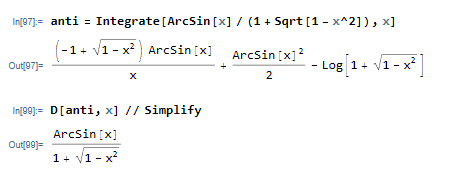I had to go back to Maple 2018, to get no exception: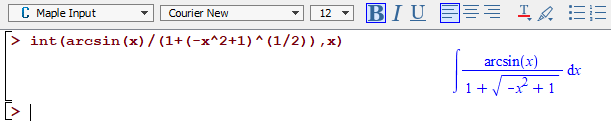May be this could be looked at for next Maple version?

## Probe Info tooltips not visible on Linux...

My PC is dual-booted (Win10 and Ubuntu 20.04+KDE). After creating a plot (even the simplest one) and enabling Probe Info as described in  https://www.maplesoft.com/support/help/maple/view.aspx?path=worksheet%2Fplotinterface%2Fpointprobei I do not see tooltip with cursor position. All other tooltips (Palettes, Toolbar etc.) work fine, it is only the probe Info tooltip that does not work. The same plot done while running Maple on Win10 works as expected - tooltip is visible.

Can someone confirm whether it works for them using Linux? I wonder whether this could be Java-related problem when running on Linux KDE with compositing and using Nvidia driver (e.g. color of tooltip is white on white, or rendering does not work as expected).

I do not remember whether it ever worked on Linux. At this moment I am using Maple2021 but for testing purposes I reinstalled Maple2020 and the problem is the same.

Thanks,

Alex

## how to prevent ExportMatrix from adding an extra \...

I have plain text file generated, where each line has mixed numbers and strings. Some of the strings are latex. So they have "\" in them. The data is rectangle. same number of fields on each line, separated by commas.  I use CSV format to read the data to Maple.

I simply want to read this file using Import , which reads it into a matrix (using CSV format), and export it back right away using either ExportMatrix or Export and end up with the same exact file.

But Maple always adds extra "\" each time I export the matrix back to the file. So if I have initially "\sin x" in the file, after reading/writing the file 5 times without touching the data inside Maple, I end up with something like "\\\\\\\\\\\sin x" in the file at the end.

I could ofcourse not use ExportMatrix nor Export and write the data back to the file manually using fprintf using correct format for each field. But it is easier if I can get ExportMatrix to work since there are many fields some are integers, some are real and some are strings.

Here is a simple MWE which shows the problem. Originally I have this file on disk. Called test.txt and has 2 lines in it

1,"\sin x"
2,"this is second line"

Now after doing the following

data:=Import("test.txt",format="CSV",output=Matrix):
ExportMatrix("test.txt",data,target=csv);


The file on disk now becomes

1,"\\sin x"
2,"this is second line"

And repeating the above one more time, the file becomes

1,"\\\\sin x"
2,"this is second line"

This ofcourse breaks all the latex strings in there.

Is there a way to prevent Maple from doing this? I looked at all the options in help, but do not see on so far.  I also tried Export but that did not work at all. So I'd like to see if ExportMatrix can do it, without adding an extra "\", otherwise, will have to use fprintf directly to export the data back to file.

edit

fyi, I ended up using fprintf to write the data back to disk. May be if there is a solution using ExportMatrix, will change back. This is what I ended up doing

File before

1,"\sin x"
2,"this is second line"

Now run this code

data:=Import("test.txt",format="CSV",output=Matrix):
file_id := fopen("test.txt",WRITE):
nRows:=LinearAlgebra:-RowDimension(data);
for n to nRows do
if n<nRows then
fprintf(file_id,"%d,\"%s\"\n",data[n,1],data[n,2]);
else
fprintf(file_id,"%d,\"%s\"",data[n,1],data[n,2]);
fi;
od;
fclose(file_id);



File after

1,"\sin x"
2,"this is second line"


Now the file is exactly the same as before reading it.

My actual data is more than the above two fields, but that makes the format string longer, that is all.

## Maple 2021.1 update

by: Maple 2021

We have just released an update to Maple, Maple 2021.1.

Maple 2021.1 includes improvements to plotting, export to PDF and LaTeX, the user interface, the mathematics engine, and more. We strongly recommend that all Maple 2021 users install these updates.

This update is available through Tools>Check for Updates in Maple, and is also available from our website on the Maple 2021.1 download page, where you can also find more details.

In particular, please note that this update includes fixes to the sometimes missing plotting toolbar, the misplaced plot annotations on export, and a workbook saving problem, all reported on MaplePrimes.

Thanks for the feedback!

## timelimit still does not work for some cases in Ma...

timelimit() has improved in Maple 2021, and I thought it works now for everything.

https://www.mapleprimes.com/maplesoftblog/213986-Introducing-Maple-Learn-officially#comment204444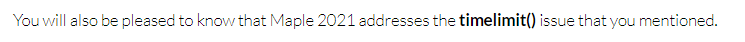But unfortunately,, it still hangs on some dsolves when I was testing something. Here is an example

restart;
ode:=(y(x)^4-a^2*x^2)*diff(y(x),x)^2+2*a^2*x*y(x)*diff(y(x),x)+y(x)^2*(y(x)^2-a^2)=0;
timelimit(60,dsolve(ode,y(x)))


The above has one minute timeout. But its been running for 20 minutes now. Here is another example that hangs

restart;
ode:=(diff(y(x),x) = (-y(x)^2+4*a*x)^3/(-y(x)^2+4*a*x-1)/y(x));
timelimit(60,dsolve(ode,y(x)))

I have found many more dsolve examples that hang with timelimit. Will update later. May be they will help find the cause.

Hopefully this timelimit issue will be fixed in some future release.  hangs in Maple commands even when using timelimit makes it very hard to run long scripts that run over many problems, since do not know when/where it will hang and have to restart and clean things manually each time.

Window 10. Maple 2021

Update March 15,2022

Here is another example of timelimit taking so much longer than asking for, it is pretty much useless.

I will use this question to collect such examples in one place. Here I asked for one minute (60 seconds, CPU time) timeout. Maple actually timedout after using 1381 CPU seconds which is 23 times as much as requested.

 > restart;
 > ode:=x^2*(x^2+1)*diff(y(x),x$2)+7*x*exp(x)*diff(y(x),x)+9*(1+tan(x))*y(x)=0; current_time:=time(); try timelimit(60,dsolve(ode)) catch: print("Good. Timelimit worked"); end try; time_used := time()-current_time;> 1381/60.> Download timelimit_example_march_15_2022.mw Help says The timelimit function evaluates the expression x, but gives up if the evaluation takes longer than the number of seconds specified by t. Note: In some cases, the execution may not abort at exactly the time limit imposed, but will abort as soon as it can do so safely. This can happen when execution is in critical sections of certain built-in routines. For me, the above makes no sense at all. What does "as soon as it can do safely"? I am not running the code to control an airplane flight full of people here. This is some code running on my PC at home to do some calculation. It can abort right away. It will not hurt me physically or hurt my PC to do so. So what does "do so safely" actually mean? Safety of what exactly? Compare the above example to Mathematica where it times out at almost exactly to the requested timeout. Here I ask for 3 seconds timeoutI am not asking for exact timeout. But asking for 1 minute timeout and getting 8 minutes is not good at all. 1.5 minutes is OK. even 2 minutes is OK. I have more examples to add. But will do that later. Update Quick try with Maple 2022 shows timelimit has much improved. Here are 3 examples from above. First one asks for 60 seconds limit, it finishes in 288 seconds. Second one asks for 60 seconds, it finishes in 96 seconds. Third one asks for 60 seconds and finishes in 73 second. This is much much better than in Maple 2021. Thanks for the Maple engineers who improved this.  > interface(version)> restart;  > ode:=x^2*(x^2+1)*diff(y(x),x$2)+7*x*exp(x)*diff(y(x),x)+9*(1+tan(x))*y(x)=0; current_time:=time(); try    timelimit(60,dsolve(ode)) catch:    print("Good. Timelimit worked"); end try; time_used := time()-current_time;> ode:=(y(x)^4-a^2*x^2)*diff(y(x),x)^2+2*a^2*x*y(x)*diff(y(x),x)+y(x)^2*(y(x)^2-a^2)=0; current_time:=time(); try    timelimit(60,dsolve(ode)) catch:    print("Good. Timelimit worked"); end try; time_used := time()-current_time;> ode:=(diff(y(x),x) = (-y(x)^2+4*a*x)^3/(-y(x)^2+4*a*x-1)/y(x)); current_time:=time(): try    timelimit(60,dsolve(ode)) catch:    print("Good. Timelimit worked"); end try; time_used := time()-current_time;>

## kernelopts('assertlevel'=2): causes problem when u...

I found a big problem.

When adding kernelopts('assertlevel'=2): which I like to have it on all the time to catch (actual) errors, but now Maple server hangs then crashes on what should be no issue at all.

This happens when I am using an object, which is used at global type. Here is a MWE

restart;

interface(warnlevel=4);
kernelopts('assertlevel'=2);

local ode_type:=module()
option object;
export ode;
end module:

A:=module()
export foo:=proc()
C:-foo();
end proc;

local B:=module()
export foo:=proc()
local ODE::ode_type;
return ODE;
end proc;
end module;

local C:=module()
export foo:=proc()
local the_ode::ode_type;  #THIS CAUSES the hang
#using the_ode::':-ode_type'; makes no difference
#DEBUG
the_ode:=B:-foo();
end proc;
end module;
end module;


There is nothing wrong with the above code as far as I can see. But now A:-foo(); crashes the Maple server.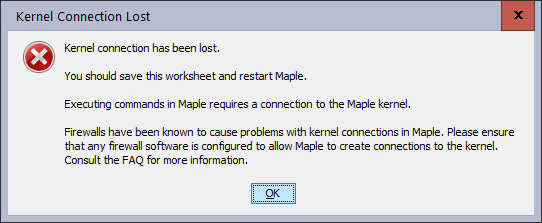If I change the line local the_ode::ode_type; to local the_ode; i.e. remove the type on the local variable, it works.

So assert thinks the type being returned is not ode_type

It also works if I remove the assert and keep the type there. Like this

restart;

interface(warnlevel=4);

local ode_type:=module()
option object;
export ode;
end module:

A:=module()
export foo:=proc()
C:-foo();
end proc;

local B:=module()
export foo:=proc()
local ODE::ode_type;
return ODE;
end proc;
end module;

local C:=module()
export foo:=proc()
local the_ode::ode_type;  #NOW IT WORKS
the_ode:=B:-foo();
end proc;
end module;
end module;

In my actual code, when I remove the kernelopts('assertlevel'=2); I do get correct final output. So it is not like I am bypassing some wrong code or something. The code works as expected.

Now now I have two choices, either remove  kernelopts('assertlevel'=2); or remove the type from the defintion of the local variables, when the type of object defined as above.

edit

another version below. When I copied the code above from my .mpl files to the worksheet to make a MWE, there was a local next to the object module (because that is how it is in my main code), and I did not notice it at first.

When I removed it to make new MWE in the worksheet, an error still shows up. But now Maple server do not hang/crash but it still does not like the assignment being made. So here is another version

restart;
interface(warnlevel=4);
kernelopts('assertlevel'=2):

ode_type:=module()  #note that local ode_type:=module()  crashes Maple.
option object;
export ode; #can be list
end module:

A:=module()
export foo:=proc()
C:-foo();
end proc;

local B:=module()
export foo:=proc()
local ODE::ode_type;
return ODE;
end proc;
end module;

local C:=module()
export foo:=proc()
local the_ode::ode_type;
#DEBUG();
the_ode:=B:-foo();
end proc;
end module;
end module;


And now A:-foo(); do not crash the server, but still gives error

Error, (in foo) assertion failed in assignment to the_ode, expected ode_type, got ODE

Why? since ODE is of type ode_type, and I am returning. Can one not return Object like this?

Is there something I am doing wrong?

## why eval(parse(command with assignment)) does not ...

is it possible for a string such as "res:=boo()" to be evaluated after calling parse, and have res have the value that the function boo() returned?

Now, it does not work. I must do   res:=eval(parse("boo()")) , meaning the result of the call to boo() is outside the string.

Here is an example of what I mean

boo:=proc()
return 99;
end proc;

foo:=proc()
local s,res,i;
s:="res:=boo()";
eval(parse(s)):
print(res);
end proc;

foo();


The above does not work. It displays res but this works

boo:=proc()
return 99;
end proc;

foo:=proc()
local s,res,i;
s:="boo()";
res:=eval(parse(s)):
print(res);
end proc;

foo();


The above works, and prints 99.

The reason I am asking, is that I was thinking of storing in a data base the complete Maple command as string, along with the LHS of the call, and just load the string the evaluate it, and it will have res automatically set.

It is no problem if this can't work, I could always just eval the command itself, without the assignment being there. Just thought to ask if there is a way.

## how to speed up development with larger code base?...

I have about 30 modules under one root common module.  The total code is about 25,000 lines.

Currently during development, when I change a line of code and want to run a test, I just do

interface(warnlevel=4);
kernelopts('assertlevel'=2):
#run some test 

From a worksheet. This works fine, but it is very slow. it takes now about 8-10 minutes to finish each time.

So each time I make small change to the source code, which all sit in separate .mpl plain text files, I have to wait and wait for the read to complete.

This is way too slow.

The thing is, my my_root_module.mpl files includes child modules (using the $include directive), and each child module might also have$include to pull in its own child modules, if any. Each module is onbe separate .mpl file.

So at the end my_root_module.mpl will end up reading all the code, which is now about 25,000 lines over 30 or so modules. Here is an example of the layout I have

my_root_module.mpl
=======
export my_root_module:=module()
$include "B.mpl"$include  "C.mpl"
$include "D.mpl" export foo:-proc().... B:-foo().... end proc; end module: B.mpl ======= local B:=module()$include  "B1.mpl"
$include "B2.mpl" export foo:=proc()..... end proc; end module: C.mpl ======= local C:=module()$include  "C1.mpl"

export foo:=proc()..... end proc;
end module:

etc...

There must be faster way to do this. Having to wait about 10 minutes each time to test one small code change each time is not practical.

What do others do when they develope large code in Maple? Is this a typical time?

I do not want to break my main modules into separate name spaces again in order to  use separate mla for each module, so that my changes only affects one mla file.  This will speed things, as I would then only have to rebuild one small modules, the one I changed.

But I want to put all my modules under one common module, which acts just as a common name space, and have only one mla file.

Are there ways to speed  reading mpl file?

Edit

Thanks to all the answers. It turned out indeed to be the slow Maple GUI again. The option interface(prettyprint= 0) did not help in the worksheet to speed the GUI.

I wrote the commands to read the mpl file and build the mla in a file, and used the DOS command line, and turned off the warning messages using DOS option, and now it finished almost instantly.

"C:\Program Files\Maple 2021\bin.X86_64_WINDOWS\cmaple.exe" UPDATE_MLA.mpl 1> nul

memory used=18.6MB, alloc=73.3MB, time=0.52

Notice, in the above 1>nul will not even display the warning messages on the terminal. 2>nul does not work, since these are not error messages. Here are the different redirection options from the net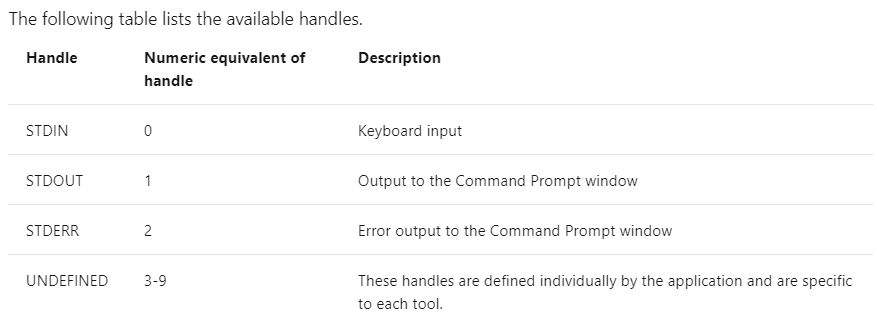But even without using this redirection, and keeping the messages scroll on the terminal, it was still very very fast from the command line

I complained about how slow the Maple Java GUI when it comes to scrolling and how it can can cause  slow down many times before, so will not complain again about. May be one day Maplesoft will fix this.

﻿